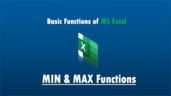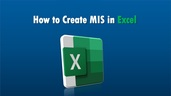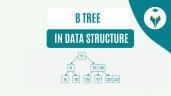Difference Between Formula and Function in Excel

# Difference Between Formula and Function in Excel

clickHere
Updated on Jul 7, 2023 17:05 IST

Formula and Function in Excel sound similar and are often confusing. The article tries to differentiate between Formula and Function.

Many people use formulas and functions in Excel interchangeably. Interestingly, these two are not the same, and even people already working on Excel are unaware of it. This article will discuss the differences between formula and function in Excel.

## Formulas in Excel – Definition

As the name suggests, a formula in Excel is a mathematical equation. It is made from values ​​or mathematical data that we have entered into the cells. You can create a formula from direct values ​​or with cell references. All formulas begin with the symbol = and then add the equation values.

Formulas must begin with an equal sign. Further, you would need to add the arguments for it to calculate and get the final expressions. Once you add a formula to a cell, you’ll see it displayed in the formula bar at the top of the sheet.

### Formulas With Functions

If you select a function that you want to use, add it to the formula, and then include arguments that can include cell references, numbers, or text values. Examples of simple formulas that use functions in Excel are:

=SUM(B2:B10)

=COUNT(B2:B10)

=AVERAGE(B2:B10)

=TRIM(B1)

### Formulas Without Functions

You can use formulas alone, without functions. This way, you can perform tasks such as adding numbers and multiplying values ​​in cells. Examples of basic formulas without functions in Excel include:

=A1+A2

=C1-C2

=2*4

=B1/B2

=D1*D2Most Popular and Powerful Formulas in Excel
The article discusses the most popular formulas in excel through practical examples of the use of formulas for Excel.Average Function in Excel – Formula and Examples
The AVERAGE function is a popular Excel function used for various purposes, such as calculating average sales, average student scores, average monthly expenses, etc. It provides a quick and efficient...read moreMIN and MAX Functions in MS Excel
Learn about MIN and MAX functions in MS Excel in this blog.

## Functions in Excel – Definition

Functions in Excel are formulas predefined within the spreadsheet; they are executed using specific values ​​known as arguments. These values have a structure and follow a particular sequence, like an Excel macro.

Unlike formulas, we cannot modify the content of functions. They perform simple calculations such as SUM or AVERAGE, but also many more complex ones.  Functions are displayed in capital letters, are available for selection and use, and can be inserted into created formulas.

Every function in Excel requires a specific syntax or composition. You can see this syntax when entering a function that helps you create the formula. For example, if you click the Insert Function (fx) icon to the left of the formula bar, you can search for a function to insert.

At the bottom of the window, you will see the required syntax for each selected function in the list.

In Functions, you can see some arguments in the syntax, like “value” or “number.” These arguments tell the function what it needs to calculate. You can use some functions without arguments. However, you can’t use functions like the SUM function. It must be placed in a formula that contains arguments enclosed in parentheses.

On the other hand, functions can also be used without arguments. For example, to insert the current date and time into a cell, you can use NOW(), a fundamental function. As you can see, there are no arguments, but parentheses accompany the function as part of the syntax.Top MS Excel Interview Questions
Master your Excel interview with confidence using our curated list of top questions. From basic functions to advanced data analysis, boost your Excel proficiency and shine in any data-driven role.Introduction to MS Excel – A Beginner’s Guide
Let’s gets started with MS Excel, the most useful tool to crunch numbers and derive information for data analysts and scientists.How to Create MIS Reports in Excel?
MIS reports are crucial to measure the business performance and contribute towards meeting the overall business goals. In this article, you will learn easy steps to create an MIS report...read more

## Difference Between Formula and Function in Excel

The main difference between a function and formula is that a function is a predefined formula available in MS Excel, while a formula is a predefined calculation that uses cell references or hard-coded numbers to calculate a cell value.

Technically, you can call a function a formula. The only difference is that a function is a predefined formula. We do not get to create functions. We can use formulas with functions.

So, the easiest way to tell the difference between a function and a formula in Excel is to insert a function into a formula you create.

Function: Predefined by Excel and can be inserted into a formula.

Formula: User-defined and can be used with or without a function.

You can create functions from mathematical formulas but cannot modify them. The program predefines them.

They have in common that both resources facilitate mathematical operations, beginning with the = symbol. But its structure is different. You can create formulas by adding values ​​and assigning the mathematical operation (+,-,*,/).

Functions are made from arguments using parentheses. A practical case would be:

Example of a formula: = A1+B1

Example of a function: =SUM(A1:B1)

Excel provides help to fill in the arguments correctly. If you miswrite any sequence, a warning pops up and offers options to correct it.

Check how Excel recommends correcting a typo in the formula in the above example. However, you would need to pay attention that you don’t make any errors while creating any formula; Excel detects typos and not any major misses.

#### Top Trending Articles in MS Excel:

clickHere

Rashmi is a postgraduate in Biotechnology with a flair for research-oriented work and has an experience of over 13 years in content creation and social media handling. She has a diversified writing portfolio and aim... Read Full Bio

## Trending Management CoursesExcel for Beginners
Great Learning4.6Microsoft Excel - Excel from Beginner to Advanced
UDEMY4.7Coursera4.7Excel to MySQL: Analytic Techniques for Business SpecializationExcel to MySQL: Analytic Techniques for Business S...
Coursera4.7Starts todayCreate Charts and Dashboards Using Microsoft Excel
Coursera4.0Starts todayUsing Basic Formulas and Functions in Microsoft ExcelUsing Basic Formulas and Functions in Microsoft Ex...
Coursera5.0Starts today

## Top Picks & New Arrivals